# derivative

Time derivative of UAV states

## Syntax

``stateDerivative = derivative(uavGuidanceModel,state,control,environment)``

## Description

example

````stateDerivative = derivative(uavGuidanceModel,state,control,environment)` determines the time derivative of the state of the UAV guidance model using the current state, control commands, and environmental inputs. Use the state and time derivative with `ode45` to simulate the UAV.```

## Examples

collapse all

This example shows how to use the `multirotor` guidance model to simulate the change in state of a UAV due to a command input.

Create the multirotor guidance model.

`model = multirotor;`

Create a state structure. Specify the location in world coordinates.

```s = state(model); s(1:3) = [3;2;1];```

Specify a control command, `u`, that specified the roll and thrust of the multirotor.

```u = control(model); u.Roll = pi/12; u.Thrust = 1;```

Create a default environment without wind.

`e = environment(model);`

Compute the time derivative of the state given the current state, control command, and environment.

`sdot = derivative(model,s,u,e);`

Simulate the UAV state using `ode45` integration. The `y` field outputs the multirotor UAV states as a 13-by-n matrix.

```simOut = ode45(@(~,x)derivative(model,x,u,e), [0 3], s); size(simOut.y)```
```ans = 1×2 13 3536 ```

Plot the change in roll angle based on the simulation output. The roll angle (the X Euler angle) is the 9th row of the `simOut.y` output.

`plot(simOut.y(9,:))`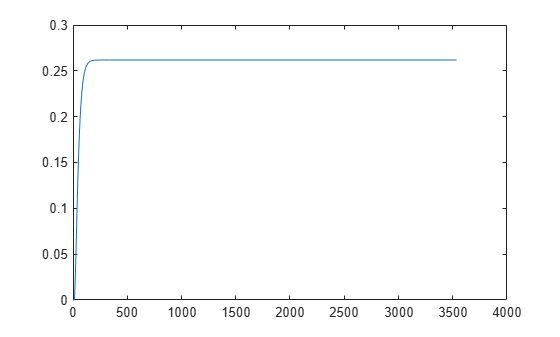Plot the change in the Y and Z positions. With the specified thrust and roll angle, the multirotor should fly over and lose some altitude. A positive value for Z is expected as positive Z is down.

```figure plot(simOut.y(2,:)); hold on plot(simOut.y(3,:)); legend('Y-position','Z-position') hold off```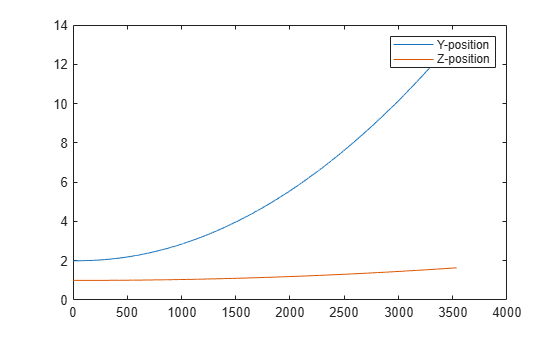You can also plot the multirotor trajectory using `plotTransforms`. Create the translation and rotation vectors from the simulated state. Downsample (every 300th element) and transpose the `simOut` elements, and convert the Euler angles to quaternions. Specify the mesh as the `multirotor.stl` file and the positive Z-direction as `"down"`. The displayed view shows the UAV translating in the Y-direction and losing altitude.

```translations = simOut.y(1:3,1:300:end)'; % xyz position rotations = eul2quat(simOut.y(7:9,1:300:end)'); % ZYX Euler plotTransforms(translations,rotations,... 'MeshFilePath','multirotor.stl','InertialZDirection',"down") view([90.00 -0.60])```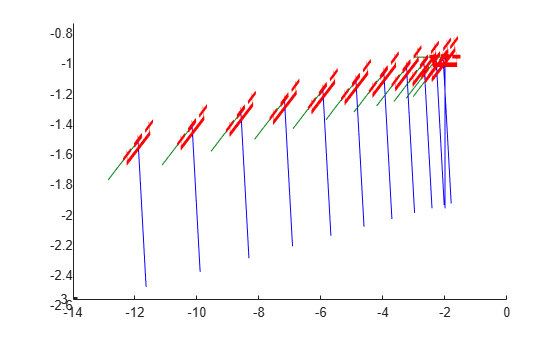This example shows how to use the `fixedwing` guidance model to simulate the change in state of a UAV due to a command input.

Create the fixed-wing guidance model.

`model = fixedwing;`

Set the air speed of the vehicle by modifying the structure from the `state` function.

```s = state(model); s(4) = 5; % 5 m/s```

Specify a control command, `u`, that maintains the air speed and gives a roll angle of `pi/12`.

```u = control(model); u.RollAngle = pi/12; u.AirSpeed = 5;```

Create a default environment without wind.

`e = environment(model);`

Compute the time derivative of the state given the current state, control command, and environment.

`sdot = derivative(model,s,u,e);`

Simulate the UAV state using `ode45` integration. The `y` field outputs the fixed-wing UAV states based on this simulation.

```simOut = ode45(@(~,x)derivative(model,x,u,e), [0 50], s); size(simOut.y)```
```ans = 1×2 8 904 ```

Plot the change in roll angle based on the simulation output. The roll angle is the 7th row of the `simOut.y` output.

`plot(simOut.y(7,:))`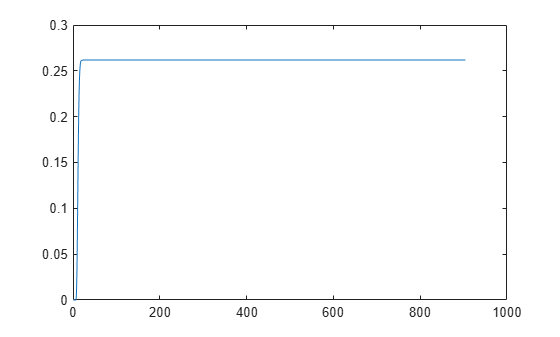You can also plot the fixed-wing trajectory using `plotTransforms`. Create the translation and rotation vectors from the simulated state. Downsample (every 30th element) and transpose the `simOut` elements, and convert the Euler angles to quaternions. Specify the mesh as the `fixedwing.stl` file and the positive Z-direction as `"down"`. The displayed view shows the UAV making a constant turn based on the constant roll angle.

```downsample = 1:30:size(simOut.y,2); translations = simOut.y(1:3,downsample)'; % xyz-position rotations = eul2quat([simOut.y(5,downsample)',simOut.y(6,downsample)',simOut.y(7,downsample)']); % ZYX Euler plotTransforms(translations,rotations,... 'MeshFilePath','fixedwing.stl','InertialZDirection',"down") hold on plot3(simOut.y(1,:),-simOut.y(2,:),simOut.y(3,:),'--b') % full path xlim([-10.0 10.0]) ylim([-20.0 5.0]) zlim([-0.5 4.00]) view([-45 90]) hold off```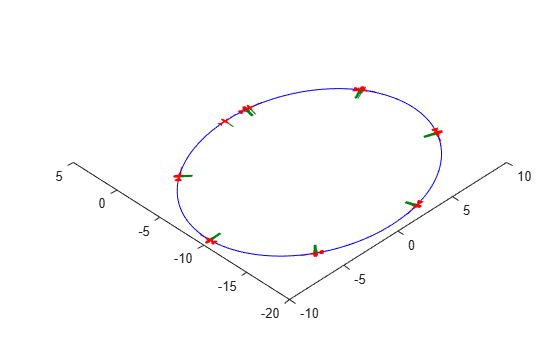## Input Arguments

collapse all

UAV guidance model, specified as a `fixedwing` or `multirotor` object.

State vector, specified as a eight-element or thirteen-element vector. The vector is always filled with zeros. Use this function to ensure you have the proper size for your state vector.

For fixed-wing UAVs, the state is an eight-element vector:

• North - Position in north direction in meters.

• East - Position in east direction in meters.

• Height - Height above ground in meters.

• AirSpeed - Speed relative to wind in meters per second.

• HeadingAngle - Angle between ground velocity and north direction in radians.

• FlightPathAngle - Angle between ground velocity and north-east plane in radians.

• RollAngle - Angle of rotation along body x-axis in radians per second.

• RollAngleRate - Angular velocity of rotation along body x-axis in radians per second.

For multirotor UAVs, the state is a thirteen-element vector in this order:

• World Position - `[x y z]` in meters.

• World Velocity - `[vx vy vz]` in meters per second.

• Euler Angles (ZYX) - `[psi theta phi]` in radians.

• Body Angular Rates - `[p q r]` in radians per second.

• Thrust - `F` in Newtons.

Environmental input parameters, returned as a structure. To generate this structure, use `environment`.

For fixed-wing UAVs, the fields of the structure are `WindNorth`, `WindEast`,`WindDown`, and `Gravity`. Wind speeds are in meters per second, and negative speeds point in the opposite direction. Gravity is in meters per second squared (default `9.81`).

For multirotor UAVs, the only element of the structure is `Gravity` (default `9.81`) in meters per second squared.

Control commands for UAV, specified as a structure. To generate this structure, use `control`.

For multirotor UAVs, the guidance model is approximated as separate PD controllers for each command. The elements of the structure are control commands:

• `Roll` - Roll angle in radians.

• `Pitch` - Pitch angle in radians.

• `YawRate` - Yaw rate in radians per second. (D = 0. P only controller)

• `Thrust` - Vertical thrust of the UAV in Newtons. (D = 0. P only controller)

For fixed-wing UAVs, the model assumes the UAV is flying under the coordinated-turn condition. The Guidance Model equations assume zero side-slip. The elements of the bus are:

• `Height` - Altitude above the ground in meters.

• `Airspeed` - UAV speed relative to wind in meters per second.

• `RollAngle` - Roll angle along body forward axis in radians. Because of the coordinated-turn condition, the heading angular rate is based on the roll angle.

## Output Arguments

collapse all

Time derivative of state, returned as a vector. The time derivative vector has the same length as the input `state`.

## Version History

Introduced in R2018b